### Algebra-Solutions Ex-11.1

CBSE Class –VI Mathematics
NCERT Solutions
Chaper 11 Algebra (Ex. 11.1)

Question 1. Find the rule, which gives the number of matchsticks required to make the following matchsticks patterns. Use a variable to write the rule.
(a) A pattern of letter T as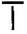(b) A pattern of letter Z as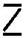(c) A pattern of letter U as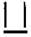(d) A pattern of letter V as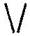(e) A pattern of letter E as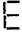(f) A pattern of letter S as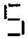(g) A pattern of letter A as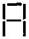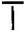$2n$ (as two matchsticks used in each letter)
(b) Pattern of letter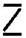$3n$ (as three matchsticks used in each letter)
(c) Pattern of letter$3n$ (as three matchsticks used in each letter)
(d) Pattern of letter$2n$ (as two matchsticks used in each letter)
(e) Pattern of letter$5n$ (as five matchsticks used in each letter)
(f) Pattern of letter$5n$ (as five matchsticks used in each letter)
(g) Pattern of letter$6n$ (as six matchsticks used in each letter)
Question 2. We already know the rule for the pattern of letter L, C and F. Some of the letters from Q.1 (given above) give us the same rule as that given by L. Which are these? Why does this happen?
Answer: The letter ‘T’ and ‘V’ that has pattern $2n,$ since 2 matchsticks are used in all these letters.
Question 3. Cadets are marching in a parade. There are 5 cadets in a row. What is the rule, which gives the number of cadets, given the number of rows? (Use $n$ for the number of rows)
Answer: Number of rows = $n$
Cadets in each row = 5
Therefore, total number of cadets = $5n$
Question 4. If there are 50 mangoes in a box, how will you write the total number of mangoes in terms of the number of boxes? (Use $b$ for the number of boxes)
Answer: Number of boxes = $b$
Number of mangoes in each box = 50
Therefore, total number of mangoes = $50b$
Question 5. The teacher distributes 5 pencils per student. Can you tell how many pencils are needed, given the number of students? (Use $s$ for the number of students)
Answer:Number of students = $s$
Number of pencils to each student = 5
Therefore, total number of pencils needed are = $5s$
Question 6. A bird flies 1 kilometer in one minute. Can you express the distance covered by the bird in terms of its flying time in minutes? (Use $t$ for flying time in minutes)
Answer: Time taken by bird = $t$ minutes
Speed of bird = 1 km per minute
Therefore, Distance covered by bird = speed x time = $1×t=t$ km
Question 7. Radha is drawing a dot Rangoli (a beautiful pattern of lines joining dots with chalk powder as in figure). She has 8 dots in a row. How many dots will her Rangoli have for $r$ rows? How many dots are there if there are 8 rows? If there are 10 rows?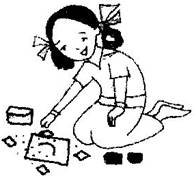Answer: Number of dots in each row = 8 dots
Number of rows = $r$
Therefore, total number of dots in r rows = $8r$
When there are 8 rows, then number of dots = 8 x 8 = 64 dots
When there are 10 rows, then number of dots = 8 x 10 = 80 dots
Question 8. Leela is Radha’s younger sister. Leela is 4 years younger than Radha. Can you write Leela’s age in terms of Radha’s age? Take Radha’s age to be $x$ years.
Answer: Radha’s age = $x$ years
Therefore, Leela’s age = $\left(x-4\right)$ years
Question 9. Mother has made laddus. She gives some laddus to guests and family members; still 5 laddus remain. If the number of laddus mother gave away is $l,$ how many laddus did she make?
Answer: Number of laddus gave away = $l$
Number of laddus remaining = 5
Total number of laddus she make = $\left(l+5\right)$
Question 10. Oranges are to be transferred from larger boxes into smaller boxes. When a large box is emptied, the oranges from it fill two smaller boxes and still 10 oranges remain outside. If the number of oranges in a small box are taken to be $x,$ what is the number of oranges in the larger box?
Answer: Number of oranges in one box = $x$
Number of boxes = 2
Therefore, total number of oranges in boxes = $2x$
Remaining oranges = 10
Thus, number of oranges = $2x+10$

Question 11. (a) Look at the following matchstick pattern of squares. The squares are not separate. Two neighbouring squares have a common matchstick. Observe the patterns and find the rule that gives the number of matchsticks in terms of the number of squares. (Hint: If you remove the vertical stick at the end, you will get a pattern of Cs.)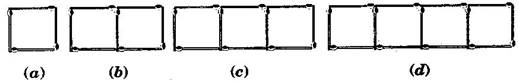(b) Figures below gives a matchstick pattern of triangles. As in Exercise 11 (a) above find the general rule that gives the number of matchsticks in terms of the number of triangles.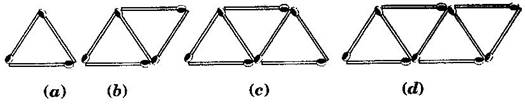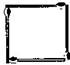4 matchsticks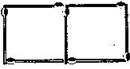7 matchsticks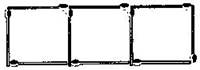10 matchsticks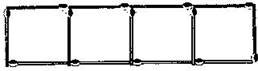13 matchsticks
If we remove 1 from each then they makes table of 3, i.e., 3, 6, 9, 12, ……….
So the required equation = $3x+1$ , where $x$ is number of squares.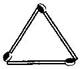3 matchsticks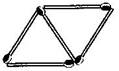7 matchsticks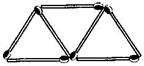10 matchsticks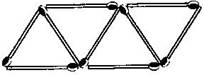13 matchsticks
If we remove 1 from each then they makes table of 2, i.e., 2, 4, 6, 8, ……….
So the required equation = $2x+1$ , where $x$ is number of triangles.